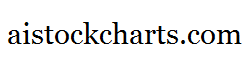Members Area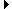Sign Up | Log In
 Free Level 2 QuotesStock AnalysisCorrelation Trading PlatformCorrelationsMembership Benefits

P3RANGE
 Lookup a different indicator.

 Indicator Usage Description Example p3range p3range[n] OPERATOR (INDICATOR or POSITIVE NUMBER) , where n is a positive integer >= 0. Returns the percentage change from the lowest price among day n, day n-1 and day n-2 to the highest price among day n, day n-1 and day n-2 where n=0 is the last trading day, n=1 is the previous day, etc. (p3range < 3)

Introduction:

The 'p3range' indicator is used in indicator formula construction to narrow the stock pre-screener results to include only those stocks that have a percentage change from the lowest to highest price of 3 consecutive days that are restricted to the parameters set for the p3range indicator.

Example:

The chart of Intel (INTC) below highlights days where the percentage change from the lowest to highest stock price of 3 consecutive days was less than 3%.

 Chart of Intel (INTC) highlighting days where the percentage change from the lowest to highest price of 3 consecutive days was less than 3%.

INDICATORS IN OUR DATABASE THAT INCLUDE THE P3RANGE INDICATOR...

Click here to view all indicators that include the indicator 'p3range' (sorted by AISCORE)

 (p3range < 3)AISCORE: 2331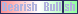Indicator ID: 060131092432(p3range < 4)AISCORE: 2696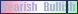Indicator ID: 060131092338(close < sma) and (close > sma) and (vol > 1.1 * vol) and (p3range < 8)AISCORE: 1046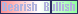Indicator ID: 060519103226(close < sma) and (close > sma) and (vol > 1.1 * vol) and (p3range < 8)AISCORE: 1644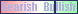Indicator ID: 060519103343(p3range < 11)AISCORE: 2197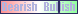Indicator ID: 060811154414 More ->